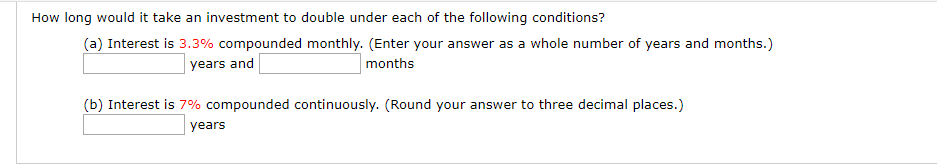# How long would it take an investment to double under each of the following conditions? (a) Interest is 3.3% compounded monthly. (Enter your answer as a whole number of years and months.) months years and (b) Interest is 7% compounded continuously. (Round your answer to three decimal places.) years

Questionhelp_outlineImage TranscriptioncloseHow long would it take an investment to double under each of the following conditions? (a) Interest is 3.3% compounded monthly. (Enter your answer as a whole number of years and months.) months years and (b) Interest is 7% compounded continuously. (Round your answer to three decimal places.) years fullscreen